Author: Oscar Cronquist Article last updated on July 09, 2022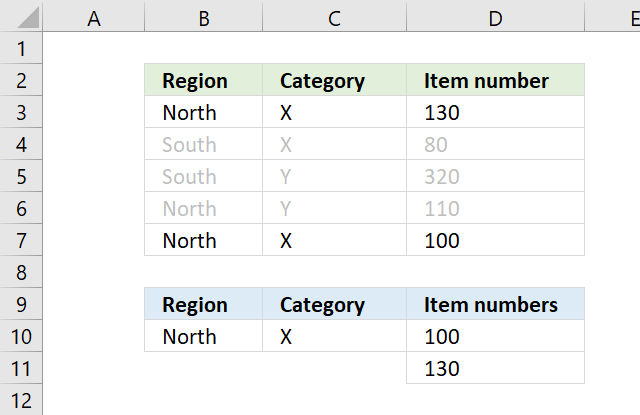This article demonstrates how to sort numbers from small to large using a condition or criteria, I will show how both AND and OR logic works, and using values and rows/records.

## 1. SMALL function - multiple conditions AND logicThe array formula in D10 extracts numbers sorted from small to large from column D if Region is equal to North AND Category is X on the same row.

=SMALL(IF((\$B\$10=\$B\$3:\$B\$7)*(\$C\$10=\$C\$3:\$C\$7), \$D\$3:\$D\$7, ""), ROWS(\$A\$1:A1))

### 1.1 How to enter an array formula

Excel 365 users can ignore these instructions, enter the formula as a regular formula. To enter an array formula, type the formula in a cell then press and hold CTRL + SHIFT simultaneously, now press Enter once. Release all keys.

The formula bar now shows the formula with a beginning and ending curly bracket telling you that you entered the formula successfully. Don't enter the curly brackets yourself.

### 1.2 Explaining formula

#### Step 1 - First condition

The equal sign compares value to value in Excel, this works fine with a value to multiple values as well. The result is either TRUE or FALSE.

\$B\$10=\$B\$3:\$B\$7

and returns

TRUE; FALSE; FALSE; TRUE; TRUE}

#### Step 2 - Second condition

\$C\$10=\$C\$3:\$C\$7

and returns

{TRUE; TRUE; FALSE; FALSE; TRUE}

#### Step 3 - Multiply arrays AND logic

There are two logical expressions in the formula returning boolean values TRUE or FALSE.

(\$B\$10=\$B\$3:\$B\$7)*(\$C\$10=\$C\$3:\$C\$7)

The asterisk between the logical expressions multiply the boolean arrays, this will help us extract numbers where both conditions match on the same row.

{TRUE; FALSE; FALSE; TRUE; TRUE}) * ({TRUE; TRUE; FALSE; FALSE; TRUE}

and returns {1; 0; 0; 0; 1}.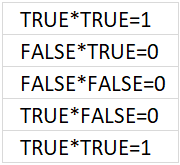The result is either 0 (zero) or 1, the numerical equivalent of TRUE is 1 and FALSE is 0 (zer0).

#### Step 4 - Replace TRUE with corresponding number on the same row

The IF function then returns the corresponding values from column D if TRUE and a blank "" if FALSE.

IF((\$B\$10=\$B\$3:\$B\$7)*(\$C\$10=\$C\$3:\$C\$7), \$D\$3:\$D\$7, "")

becomes

IF({1; 0; 0; 0; 1}, {130; 80; 320; 110; 100}, "")

and returns

{130; ""; ""; ""; 100}.

#### Step 5 - Extract k-th smallest number

The SMALL function returns the k-tk smallest value the array determined by ROWS(\$A\$1:A1).

SMALL(IF((\$B\$10=\$B\$3:\$B\$7)*(\$C\$10=\$C\$3:\$C\$7), \$D\$3:\$D\$7, ""), ROWS(\$A\$1:A1))

becomes

SMALL({130; ""; ""; ""; 100}, ROWS(\$A\$1:A1))

The cell reference in ROWS(\$A\$1:A1) expands when you copy the cell and paste it to cells below.

The smallest value is shown in D10, ROWS(\$A\$1:A1) returns 1. The second smallest value is shown in D11, ROWS(\$A\$1:A2) returns 2.

The ROWS function has a great advantage, it won't break the formula if you insert or delete rows above the array formula.

SMALL({130; ""; ""; ""; 100}, ROWS(\$A\$1:A1))

becomes

SMALL({130; ""; ""; ""; 100}, 1)

and returns 100 in cell D10.

## 2. SMALL function - multiple conditions OR logic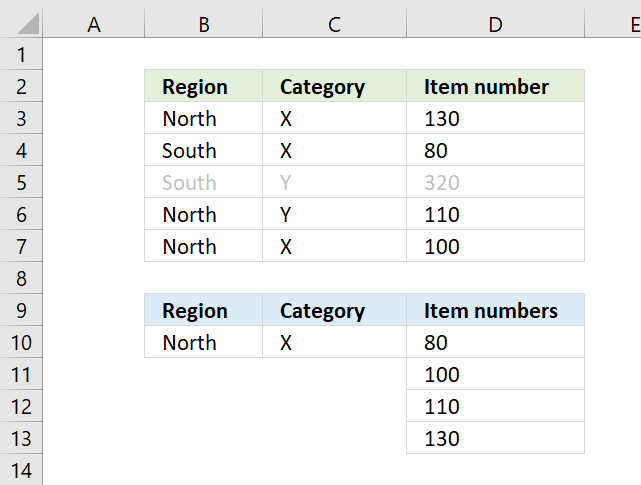The array formula in D10 extracts numbers sorted from small to large from column D if Region is equal to North OR Category is X.

=SMALL(IF((\$B\$10=\$B\$3:\$B\$7)+(\$C\$10=\$C\$3:\$C\$7), \$D\$3:\$D\$7, ""), ROWS(\$A\$1:A1))

How to enter an array formula

### Explaining formula

#### Step 1 - First condition

The equal sign compares value to value in Excel, this works fine with a value to multiple values as well. The result is either TRUE or FALSE.

\$B\$10=\$B\$3:\$B\$7

becomes

"North"={"North"; "South"; "South"; "North"; "North"}

and returns

{TRUE; FALSE; FALSE; TRUE; TRUE}.

#### Step 2 - Second condition

\$C\$10=\$C\$3:\$C\$7

becomes

"X"={"X"; "X"; "Y"; "Y"; "X"}

and returns

{TRUE; TRUE; FALSE; FALSE; TRUE}.

#### Step 3 - Add arrays

The plus sign lets you add a number with another number, you can also add arrays as long as they contain the same number of values.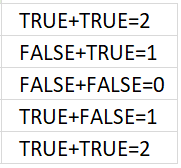If you add the logical expressions you get what the image above shows. All numbers except 0 (zero) equal TRUE and 0 (zero) is FALSE.

(\$B\$10=\$B\$3:\$B\$7)+(\$C\$10=\$C\$3:\$C\$7)

becomes

{TRUE; FALSE; FALSE; TRUE; TRUE} + {TRUE; TRUE; FALSE; FALSE; TRUE}

and returns {2; 1; 0; 1; 2}.

#### Step 4 - Replace TRUE with corresponding number on the same row

The IF function then returns the corresponding values from column D if TRUE and a blank "" if FALSE.

IF(logical_test, [value_if_true], [value_if_false])

IF((\$B\$10=\$B\$3:\$B\$7)+(\$C\$10=\$C\$3:\$C\$7), \$D\$3:\$D\$7, "")

becomes

IF({2; 1; 0; 1; 2}, \$D\$3:\$D\$7, "")

becomes

IF({2; 1; 0; 1; 2}, {130; 80; 320; 110; 100}, "")

and returns

{130; 80; ""; 110; 100}.

#### Step 5 - Extract k-th smallest number

The SMALL function returns the k-tk smallest value the array determined by ROWS(\$A\$1:A1).

SMALL(array, k)

SMALL(IF((\$B\$10=\$B\$3:\$B\$7)+(\$C\$10=\$C\$3:\$C\$7), \$D\$3:\$D\$7, ""), ROWS(\$A\$1:A1))

becomes

SMALL({130; 80; ""; 110; 100}, ROWS(\$A\$1:A1))

becomes

SMALL({130; 80; ""; 110; 100}, 1)

and returns 80.

## 3. SMALL function - match records AND logic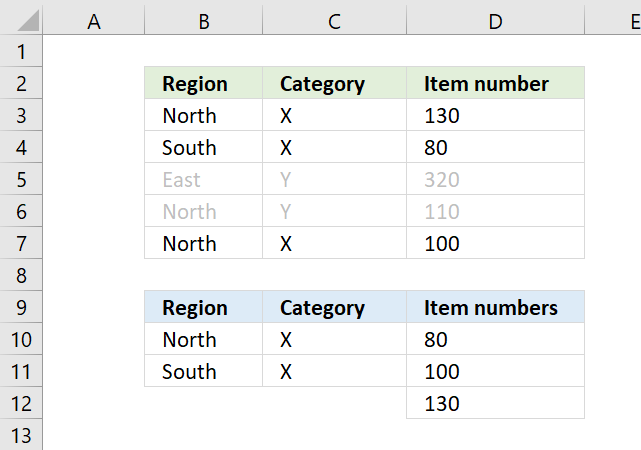The formula above in D10 extracts numbers sorted from small to large if both Region and Category match on the same row in both tables.

This formula is similar to the first formula, however, the COUNTIFS function allows you to easily use multiple criteria without building huge formulas.

=SMALL(IF(COUNTIFS(\$B\$10:\$B\$11,\$B\$3:\$B\$7, \$C\$10:\$C\$11,\$C\$3:\$C\$7), \$D\$3:\$D\$7, ""), ROWS(\$A\$1:A1))

How to enter an array formula

### 3.1 Explaining formula

#### Step 1 - Find matching rows

The COUNTIFS function calculates the number of cells across multiple ranges that equals all given conditions.

COUNTIFS(criteria_range1, criteria1, [criteria_range2, criteria2]…)

COUNTIFS(\$B\$10:\$B\$11,\$B\$3:\$B\$7, \$C\$10:\$C\$11,\$C\$3:\$C\$7)

returns {1; 1; 0; 0; 1}.

#### Step 2 - Replace any number except zero with corresponding number on the same row

The IF function then returns the corresponding values from column D if TRUE and a blank "" if FALSE.

IF(logical_test, [value_if_true], [value_if_false])

TRUE - any number except zero
FALSE - 0 (zero)

IF(COUNTIFS(\$B\$10:\$B\$11,\$B\$3:\$B\$7, \$C\$10:\$C\$11,\$C\$3:\$C\$7), \$D\$3:\$D\$7, "")

becomes

IF({1; 1; 0; 0; 1}, \$D\$3:\$D\$7, "")

becomes

IF({1; 1; 0; 0; 1}, {130; 80; 320; 110; 100}, "")

and returns {130; 80; ""; ""; 100}.

#### Step 3 - Extract k-th smallest number

The SMALL function returns the k-tk smallest value the array determined by ROWS(\$A\$1:A1).

SMALL(array, k)

SMALL(IF(COUNTIFS(\$B\$10:\$B\$11,\$B\$3:\$B\$7, \$C\$10:\$C\$11,\$C\$3:\$C\$7), \$D\$3:\$D\$7, ""), ROWS(\$A\$1:A1))

becomes

SMALL({130; 80; ""; ""; 100}, ROWS(\$A\$1:A1))

becomes

SMALL({130; 80; ""; ""; 100}, 1)

and returns 80 in cell D10.

## 4. SMALL function - match records OR logic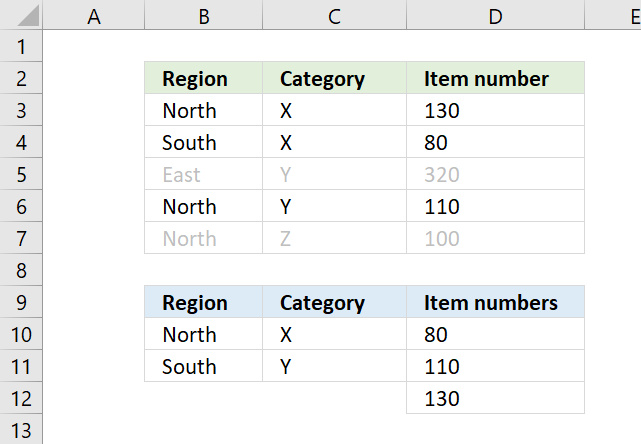The formula above in D10 extracts numbers sorted from small to large if both Region and Category match any of the criteria.

Example, Z on row 7 is not a match to any of the Category values in the lower table, both values must match.

=SMALL(IF(COUNTIF(\$B\$10:\$B\$11, \$B\$3:\$B\$7)*COUNTIF(\$C\$10:\$C\$11, \$C\$3:\$C\$7), \$D\$3:\$D\$7, ""), ROWS(\$A\$1:A1))

How to enter an array formula

### 4.1 Explaining formula

#### Step 1 - Count cells based on criteria in B10 and B11

The COUNTIF function calculates the number of cells that is equal to a condition.

COUNTIF(rangecriteria)

COUNTIF(\$B\$10:\$B\$11, \$B\$3:\$B\$7)

becomes

COUNTIF({"North"; "South"},{"North"; "South"; "East"; "North"; "North"})

and returns {1; 1; 0; 1; 1}.

#### Step 2 - Count cells based on criteria in C10 and C11

COUNTIF(\$C\$10:\$C\$11, \$C\$3:\$C\$7)

becomes

COUNTIF({"X"; "Y"},{"North"; "South"; "East"; "North"; "North"})

and returns {1; 1; 1; 1; 0}.

#### Step 3 - Multiply arrays

Multiply the arrays to create AND logic, here is how it works:

1 * 1 = 1 (TRUE)
1*0 = 0 (FALSE)
0*0 = 0 (FALSE)

COUNTIF(\$B\$10:\$B\$11, \$B\$3:\$B\$7)*COUNTIF(\$C\$10:\$C\$11, \$C\$3:\$C\$7)

becomes

{1; 1; 0; 1; 1} * {1; 1; 1; 1; 0}

and returns {1; 1; 0; 1; 0}.

#### Step 4 - REPLACE 1 (TRUE) with corresponding number on the same row

The IF function then returns the corresponding values from column D if TRUE and a blank "" if FALSE.

IF(logical_test, [value_if_true], [value_if_false])

IF(COUNTIF(\$B\$10:\$B\$11, \$B\$3:\$B\$7)*COUNTIF(\$C\$10:\$C\$11, \$C\$3:\$C\$7), \$D\$3:\$D\$7, "")

becomes

IF({1; 1; 0; 1; 0}, \$D\$3:\$D\$7, "")

becomes

IF({1; 1; 0; 1; 0}, {130; 80; 320; 110; 100}, "")

and returns {130; 80; ""; 110; ""}.

#### Step 5 - Extract k-th smallest number

The SMALL function returns the k-tk smallest value the array determined by ROWS(\$A\$1:A1).

SMALL(array, k)

SMALL(IF(COUNTIF(\$B\$10:\$B\$11, \$B\$3:\$B\$7)*COUNTIF(\$C\$10:\$C\$11, \$C\$3:\$C\$7), \$D\$3:\$D\$7, ""), ROWS(\$A\$1:A1))

becomes

SMALL({130; 80; ""; 110; ""}, ROWS(\$A\$1:A1))

becomes

SMALL({130; 80; ""; 110; ""}, 1)

and returns 80.

## 5. SMALL function - match multiple values OR logic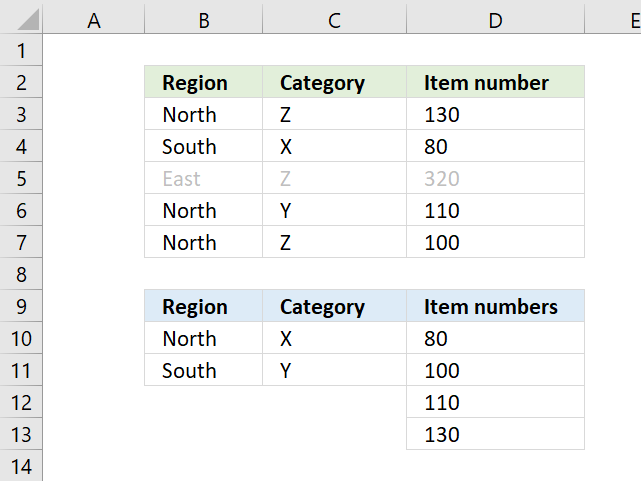The formula above in D10 extracts numbers sorted from small to large if any of the Region or Category values match.

=SMALL(IF(COUNTIF(\$B\$10:\$B\$11, \$B\$3:\$B\$7)+ COUNTIF(\$C\$10:\$C\$11, \$C\$3:\$C\$7), \$D\$3:\$D\$7, ""), ROWS(\$A\$1:A1))

How to enter an array formula

### 5.1 Explaining formula

#### Step 1 - Count cells based on criteria in B10 and B11

The COUNTIF function calculates the number of cells that is equal to a condition.

COUNTIF(rangecriteria)

COUNTIF(\$B\$10:\$B\$11, \$B\$3:\$B\$7)

becomes

COUNTIF({"North"; "South"}, {"North"; "South"; "East"; "North"; "North"})

and returns {1; 1; 0; 1; 1}.

#### Step 2 - Count cells based on criteria in C10 and C11

COUNTIF(\$C\$10:\$C\$11, \$C\$3:\$C\$7)

becomes

COUNTIF({"X"; "Y"},{"Z"; "X"; "Z"; "Y"; "Z"})

and returns {0; 1; 0; 1; 0}.

#### Step 3 - Add arrays

The plus sign lets you add numbers in an Excel formula, this works fine with arrays as well.

COUNTIF(\$B\$10:\$B\$11, \$B\$3:\$B\$7)+ COUNTIF(\$C\$10:\$C\$11, \$C\$3:\$C\$7)

becomes

{1; 1; 0; 1; 1} + {0; 1; 0; 1; 0}

and returns {1; 2; 0; 2; 1}.

#### Step 4 - Replace 1 (True) with corresponding number on the same row

The IF function then returns the corresponding values from column D if TRUE and a blank "" if FALSE.

IF(logical_test, [value_if_true], [value_if_false])

IF(COUNTIF(\$B\$10:\$B\$11, \$B\$3:\$B\$7)+ COUNTIF(\$C\$10:\$C\$11, \$C\$3:\$C\$7), \$D\$3:\$D\$7, "")

becomes

IF({1; 2; 0; 2; 1}, \$D\$3:\$D\$7, "")

becomes

IF({1; 2; 0; 2; 1}, {130; 80; 320; 110; 100}, "")

and returns {130; 80; ""; 110; 100}.

#### Step 5 - Extract k-th smallest number

The SMALL function returns the k-tk smallest value the array determined by ROWS(\$A\$1:A1).

SMALL(array, k)

SMALL(IF(COUNTIF(\$B\$10:\$B\$11, \$B\$3:\$B\$7)+ COUNTIF(\$C\$10:\$C\$11, \$C\$3:\$C\$7), \$D\$3:\$D\$7, ""), ROWS(\$A\$1:A1))

becomes

SMALL({130; 80; ""; 110; 100}, ROWS(\$A\$1:A1))

becomes

SMALL({130; 80; ""; 110; 100}, 1)

and returns 80 in cell D10.